|

# Time-Temperature-Transformation (TTT ) Diagram

T (Time) T(Temperature) T(Transformation) diagram is a plot of temperature versus the logarithm of time for a steel alloy of definite composition. It is used to determine when transformations begin and end for an isothermal (constant temperature) heat treatment of a previously austenitized alloy. When austenite is cooled slowly to a temperature below LCT (Lower Critical Temperature), the structure that is formed is Pearlite. As the cooling rate increases, the pearlite transformation temperature gets lower.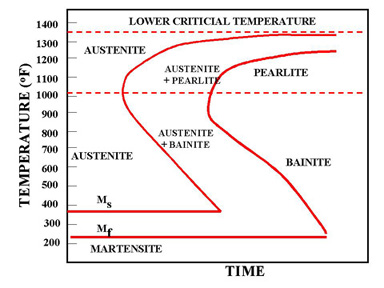Figure 1a. TTT Diagram

In Figure 1a the area on the left of the transformation curve represents the austenite region. Austenite is stable at temperatures above LCT but unstable below LCT. Left curve indicates the start of a transformation and right curve represents the finish of a transformation. The area between the two curves indicates the transformation of austenite to different types of crystal structures. (Austenite to pearlite, austenite to martensite, austenite to bainite transformation.)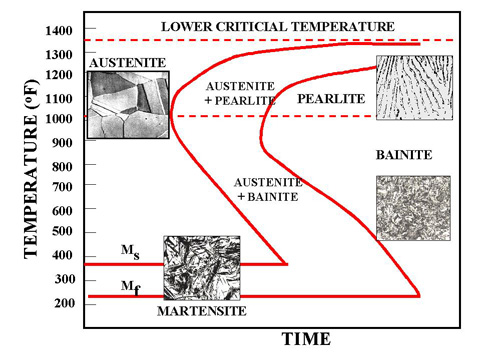##### Figure 1b. TTT Diagram and microstructures obtained by different types of cooling rates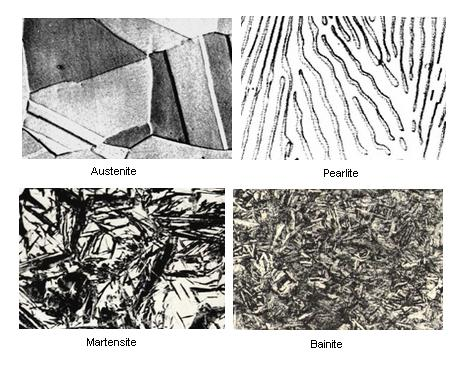##### Figure 1c. TTT Diagram and microstructures result

The microstructure of the material is significantly altered as the cooling rate increases. By heating and cooling a series of samples, the history of the austenite transformation may be recorded. TTT diagram indicates when a specific transformation starts and ends and it also shows what percentage of transformation of austenite at a particular temperature is achieved. Look at figure 1b and 1c.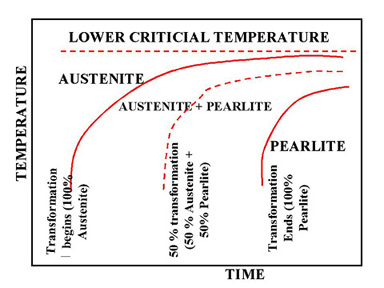##### Figure 2. Upper half of TTT Diagram(Austenite-Pearlite Transformation Area)

Figure 2 represents the upper half of the TTT diagram. As indicated in Figure 2, when austenite is cooled to temperatures below LCT, it transforms to other crystal structures due to its unstable nature. A specific cooling rate may be chosen so that the transformation of austenite can be 50 %, 100 % etc. If the cooling rate is very slow such as annealing process, the cooling curve passes through the entire transformation area and the end product of this the cooling process becomes 100% Pearlite. In other words, when slow cooling is applied, all the Austenite will transform to Pearlite. If the cooling curve passes through the middle of the transformation area, the end product is 50 % Austenite and 50 % Pearlite, which means that at certain cooling rates we can retain part of the Austenite, without transforming it into Pearlite.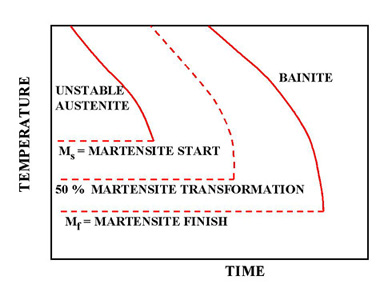##### Figure 3. Lower half of TTT Diagram (Austenite-Martensite and Bainite Transformation Areas)

Figure 3 indicates the types of transformation that can be found at higher cooling rates. If a cooling rate is very high, the cooling curve will remain on the left hand side of the Transformation Start curve. In this case all Austenite will transform to Martensite. If there is no interruption in cooling the end product will be martensite.

#####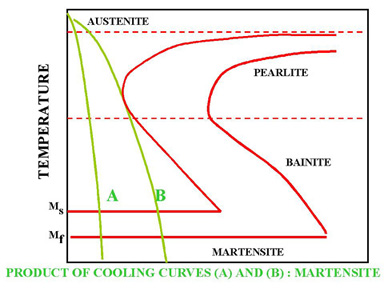Figure 4. Rapid Quench

In Figure 4 the cooling rates A and B indicate two rapid cooling processes. In this case curve A will cause a higher distortion and a higher internal stresses than the cooling rate B. The end product of both cooling rates will be martensite. Cooling rate B is also known as the Critical Cooling Rate, which is represented by a cooling curve that is tangent to the nose of the TTT diagram. Critical Cooling Rate is defined as the lowest cooling rate which produces 100% Martensite while minimizing the internal stresses and distortions.

#####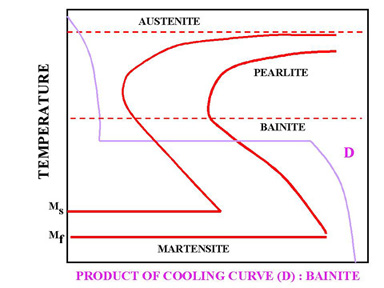Figure 5. Interrupted Quench

In Figure 5, a rapid quenching process is interrupted (horizontal line represents the interruption) by immersing the material in a molten salt bath and soaking at a constant temperature followed by another cooling process that passes through Bainite region of TTT diagram. The end product is Bainite, which is not as hard as Martensite. As a result of cooling rate D; more dimensional stability, less distortion and less internal stresses are created.

#####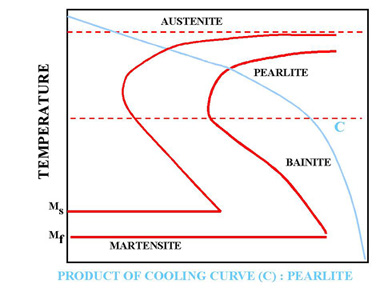Figure 6. Slow cooling process (Annealing)

In Figure 6 cooling curve C represents a slow cooling process, such as furnace cooling. An example for this type of cooling is annealing process where all the Austenite is allowed to transform to Pearlite as a result of slow cooling.

#####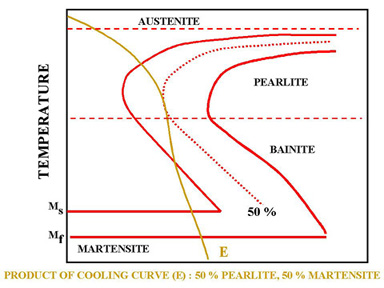Figure 7. Cooling rate that permits both pearlite and martensite formation.

Sometimes the cooling curve may pass through the middle of the Austenite-Pearlite transformation zone. In Figure 7, cooling curve E indicates a cooling rate which is not high enough to produce 100% martensite. This can be observed easily by looking at the TTT diagram. Since the cooling curve E is not tangent to the nose of the transformation diagram, austenite is transformed to 50% Pearlite (curve E is tangent to 50% curve). Since curve E leaves the transformation diagram at the Martensite zone, the remaining 50 % of the Austenite will be transformed to Martensite.

### Random Posts

• Phase Diagram of Steel
The diagram shows iron and carbons combined to form Fe-Fe3C at the 6.67%C end of the diagram. The left side of the diagr...
• Inclusions Phenomena at Steelmaking
One tonne of steel, a cube with sides of about 0,5m, contains between 1012 and 1015 inclusions which can occupy up to ab...
• Futuristic Materials part 2
Aerogel holds 15 entries in the Guinness Book of Records, more than any other material....